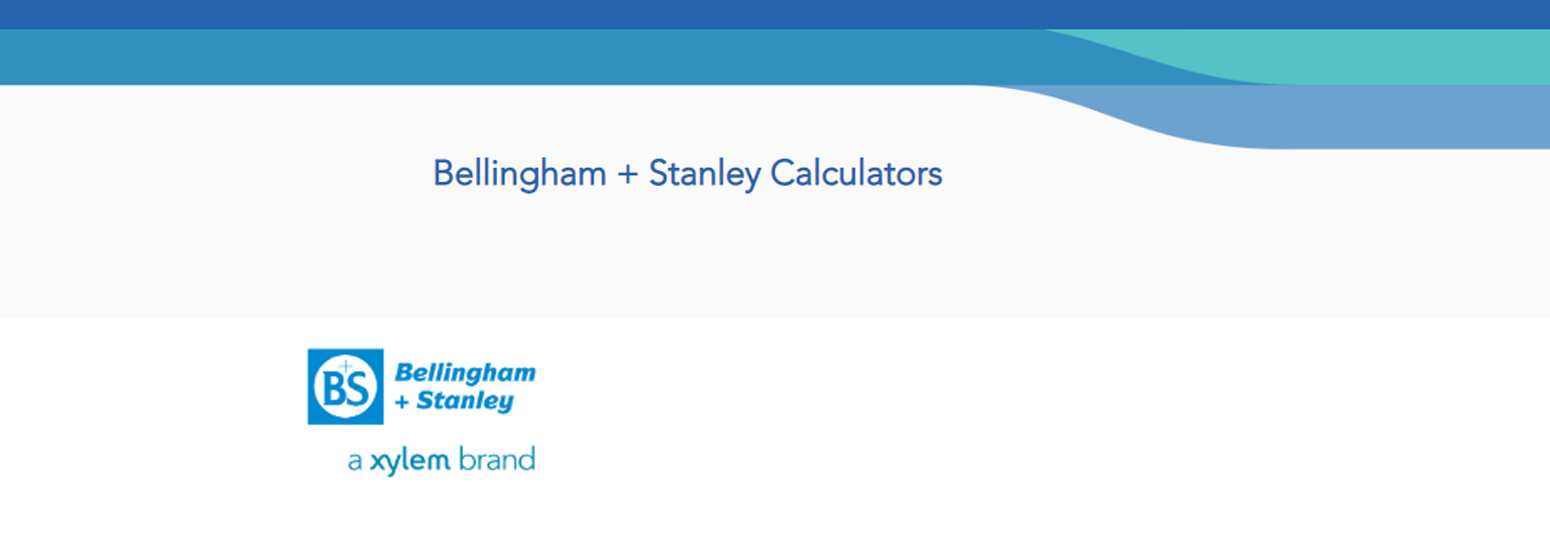# Bellingham&Stanley Refractometry Calculators vol2

## Calibration Oil Refractive Index and °Brix Calculator

B+S Ltd. Refractometer Calibration Fluids – Calibration Oil R.I. Calculator

Refractive Index values are calculated from a data fit of empirical results, which is valid over the range 10 to 75°C. The values used to determine this relationship were produced from a statistical evaluation of averaged refractive index data measured on a high accuracy refractometer at 589.3nm. °Brix values are equivalent scale values in accordance with the relationship between Refractive Index and °Brix published by ICUMSA. Refractive Index and °Brix values will only be displayed if the temperature is within the stated range.

Check the calculator here